# Mean, Median, Mode and Range Averages : Lessons and Worksheets

All | Maths | 11-16

## Learning Objectives

1. Know how data is collected and analysed in a sporting context.
2. Know what the mean, median, mode and range averages are and identify when and where you would use them.
3. Describe the difference between mean, median, mode and range averages.
4. Demonstrate and interpret the use of mean, median, mode and range averages from team data shown in charts and tables.

## Lesson Description

This Maths topic uses the real-life context of the British America’s Cup team INEOS TEAM UK, to show the crucial role of data collection and analysis in developing the performance of both the boat and crew. Students will use data generated by the team to develop their learning of mean, median, mode and range of averages. The three differentiated worksheets reinforce learning objectives for this topic. The resources can be used either in sequence or flexibly as an introduction to a topic, or for a quick activity.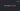This film shows how INEOS TEAM UK collected data using state-of-the-art technology to monitor and improve their performance.

## Rating

(17)For immediate access to all of our free Key stage 3 and 4 STEM teaching resources.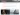2.Video Transcript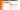3.Worksheet 1 Explore the use of weight, speed and weather data. An introduction suggested for 11-14 year olds.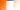4.Worksheet 1 Answers Answers to Worksheet 1.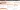5.Worksheet 2 Explore the use of weight, waste and wind data. More advanced tasks suggested for 11-14 year olds.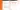6.Worksheet 2 Answers Answers to Worksheet 2.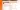7.Worksheet 3 Explore the use of data from solar panels. Suggested for 14-16 year olds.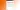8.Worksheet 3 Answers Answers to Worksheet 3.

ENGLAND
KS3 NC Mathematics – statistics
Describe, interpret and compare observed distributions of a single variable through: appropriate graphical representation involving discrete, continuous and grouped data; and appropriate measures of central tendency (mean, mode, median) and spread (range, consideration of outliers).

GCSE Mathematics
Edexcel
S2 interpret and construct tables, including frequency tables.
S4 interpret, analyse and compare the distributions of data sets from univariate empirical distributions through: appropriate graphical representation involving discrete, continuous and grouped data; appropriate measures of central tendency (median, mean, mode and modal class) and spread (range, including consideration of outliers).

AQA
S3.3, S3.3h Calculate median, mean, range, mode and modal class from lists and tables of data.
S4.4 Compare distributions and make inferences.

OCR
D2.2, D3.3 Calculate the mean, median, mode and range of discrete data.
D5.2 Use and interpret the statistical measures: mode, median, mean and range for discrete and continuous data, including comparing distributions.
D6.3 Identify the modal class of grouped data. Calculate the mean of grouped discrete data.
D7.2 Calculate the mean from grouped continuous data.

WALES
KS3 Mathematics

Find the mean, median, mode and range from grouped frequency tables.
Find the mean, median, mode and range from ungrouped frequency tables and explain why it is an estimate.
Use mean, median, mode and range to compare two distributions (continous and discrete data).
Interpret diagrams and graphs to compare sets of data.
Examine results critically, select and justify choice of statistics recognising the limitations of any assumptions and their effect on the conclusions drawn.

KS4 Mathematics
Use the mean, median, mode and range from grouped frequency tables to compare distributions.
Calculate the upper quartile, lower quartile and interquartile range of a set of discrete data and use them to describe a data set.
Recognise and use the most appropriate data to compare distributions.

SCOTLAND
Fourth level Numeracy & Mathematics:
Compare numerical information in real life contexts by finding the mean, median, mode andrange of sets of numbers. Decide which type of average is most appropriate to use and discuss how using an alternative type of average could be misleading.
Select appropriately from a wide range of tables, charts, diagrams and graphs when displaying discrete, continuous or grouped data to clearly communicate the significant features of the data.

NORTHERN IRELAND
KS3 Using Mathematics

Collect and record discrete and continuous data using a variety of methods;
Construct and interpret a variety of diagrams and graphs for discrete and continuous data;
Work out and use the median and mode;
Work out the mean, median and mode of a frequency distribution;
Use one of the measures of average to compare two sets of data.

GCSE Maths
Calculate median, mean, range and mode, and understand their uses;
Interpret a wide range of graphs and diagrams and draw conclusions.

All, Maths

11-16

### Resource For

Students, Teachers

### Type

Film, Interactive Quiz, Worksheets

### Topic

Averages and range

Please login or register to discuss these resources.